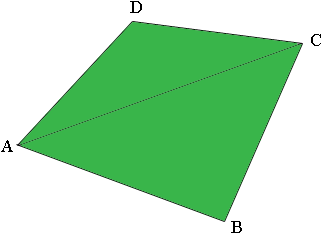SEARCH HOMEMath Central Quandaries & QueriesQuestion from Rajat: calculate the area ABCD in which AB is 48'1'',BC is 98'4'',CD is 61'4'',DA is102'10'',AC is 110'3'',BD is 116'9''.Hi Rajat,

First you should convert all the dimensions to inches. I drew a diagram (not to scale).My diagram shows the quadrilateral partitioned into two triangles and hence the area of the quadrilateral is the sum of the areas of the triangles. You know the lengths of the sides of the triangles so you can find their areas using Heron's Formula. When you are finished you should probably divide the area by $12 \times 12$ to express it in square feet.

PennyMath Central is supported by the University of Regina and The Pacific Institute for the Mathematical Sciences.